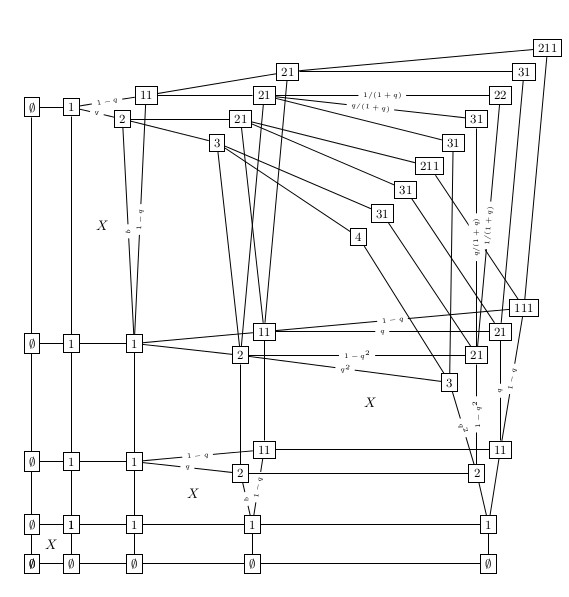Please join me to support the FSF for a free society:

# Symmetry property of $$q$$-weighted Robinson-Schensted algorithms and branching algorithms Published on 2014-04-01

In this paper a symmetry property analogous to the well known symmetry property of the normal Robinson-Schensted algorithm has been shown for the $$q$$-weighted Robinson-Schensted algorithm. The proof uses a generalisation of the growth diagram approach introduced by Fomin. This approach, which uses "growth graphs", can also be applied to a wider class of insertion algorithms which have a branching structure.Figure 1: Growth graph of q-RS for 1423

Above is the growth graph of the $$q$$-weighted Robinson-Schensted algorithm for the permutation $${1 2 3 4\choose1 4 2 3}$$.